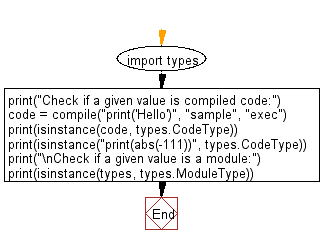﻿ Python: Check if a given value is compiled code, module - w3resource# Python: Check if a given value is compiled code, module

## Python module: Exercise-4 with Solution

Write a Python program to check if a given value is compiled code or not. Also check if a given value is a module or not. Use types.CodeType, types.ModuleType()

Sample Solution:

Python Code:

``````import types
print("Check if a given value is compiled code:")
code = compile("print('Hello')", "sample", "exec")
print(isinstance(code, types.CodeType))
print(isinstance("print(abs(-111))", types.CodeType))
print("\nCheck if a given value is a module:")
print(isinstance(types, types.ModuleType))
```
```

Sample Output:

```Check if a given value is compiled code:
True
False

Check if a given value is a module:
True
```

Flowchart:## Visualize Python code execution:

The following tool visualize what the computer is doing step-by-step as it executes the said program:

Python Code Editor:

Have another way to solve this solution? Contribute your code (and comments) through Disqus.

What is the difficulty level of this exercise?

Test your Python skills with w3resource's quiz

﻿

## Python: Tips of the Day

For-else construct useful when searched for something and find it:

```# For example assume that I need to search through a list and process each item until a flag item is found and
# then stop processing. If the flag item is missing then an exception needs to be raised.

for i in mylist:
if i == theflag:
break
process(i)
else:
raise ValueError("List argument missing terminal flag.")
```